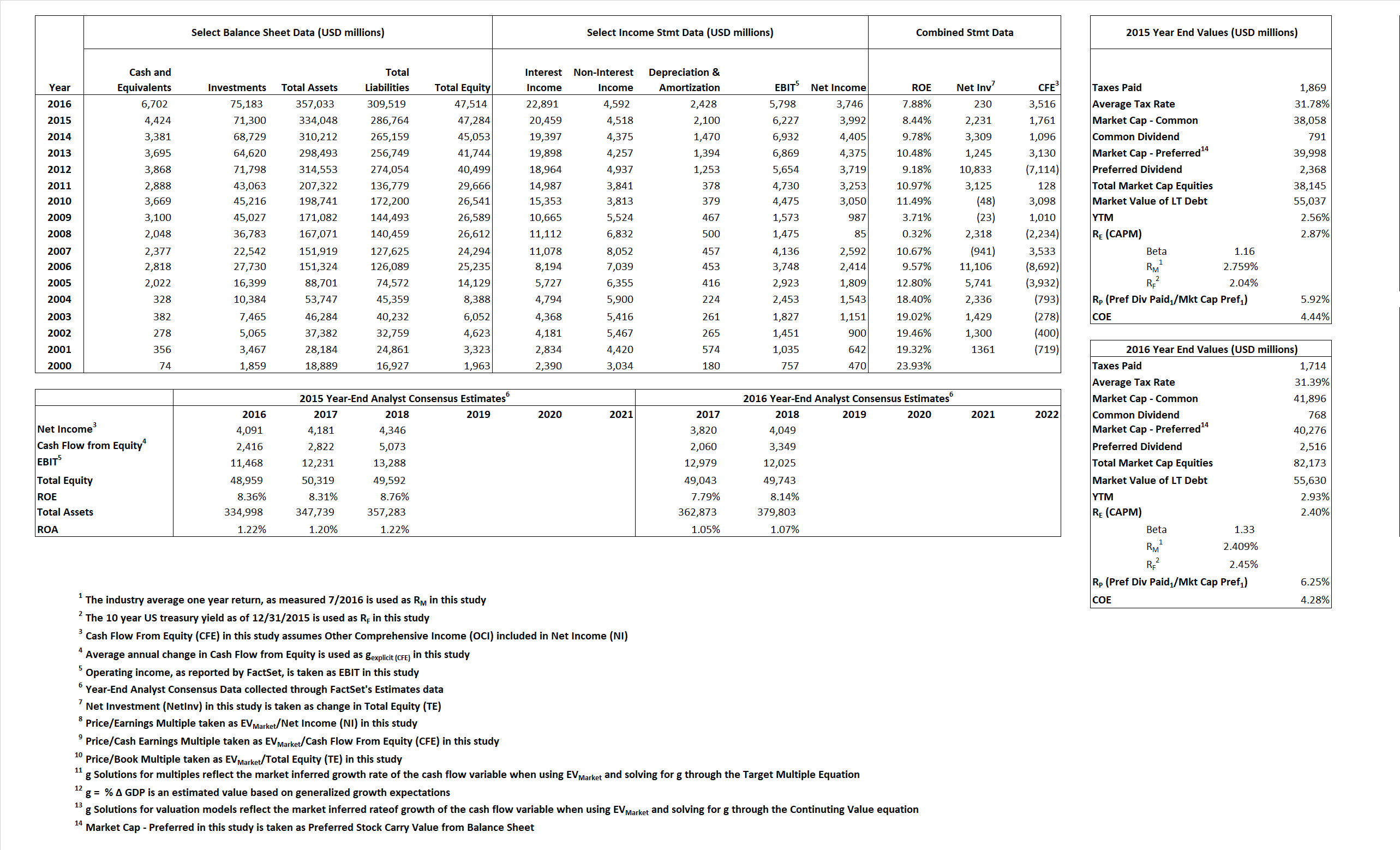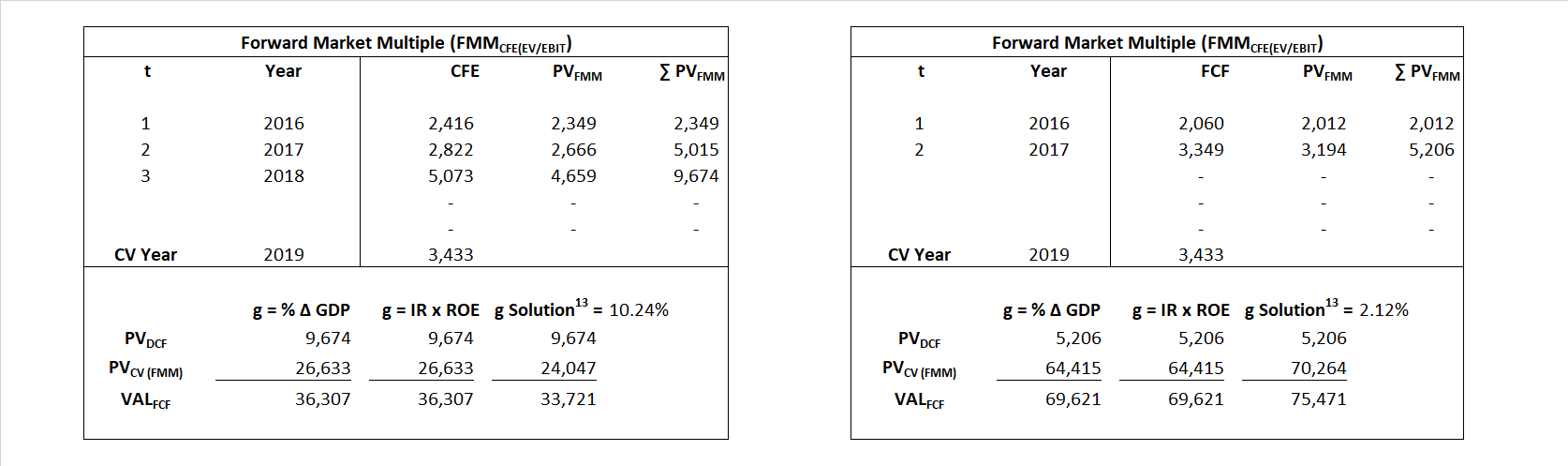# Capital One

## Analyst Listing

The following analysts provide coverage for the subject firm as of May 2016:

 Guggenheim Securities Tony Butler tony.butler@guggenheimpartners.com Credit Suisse Vamil Divan vamil.divan@credit-suisse.com Wells Fargo Securities Andrew M. Casey andrew.casey@wellsfargo.com Oppenheimer Ben Chittenden cdonat@sandleroneill.com Stifel Nicolaus Christopher C. Brendler ccbrendler@stifel.com Sandler O’Neill & Partners Christopher R. Donat cdonat@sandleroneill.com Drexel Hamilton David Hilder dhilder@drexelhamilton.com Evercore ISI David Raso draso@isigrp.com Longbow Research Eli Lustgarten elustgarten@longbowresearch.com BMO Capital Markets James Fotheringham james.fotheringham@bmo.com RBC Capital Markets Jason Arnold jason.arnold@rbccm.com BMO Capital Markets Joel Tiss joel.tiss@bmo.com Jefferies John Hecht jhecht@jefferies.com Evercore ISI John Pancari john.pancari@evercoreisi.com Bernstein Research Kevin St. Pierre kevin.st-pierre@bernstein.com William Blair Lawrence T. De Maria ldemaria@williamblair.com Credit Suisse Moshe Orenbuch moshe.orenbuch@credit-suisse.com Atlantic Equities Richard Radbourne r.radbourne@atlantic-equities.com Keefe Bruyette & Woods Sanjay Sakhrani ssakhrani@kbw.com DA Davidson Arren Cyganovich acyganovich@dadco.com Oppenheimer Ben Chittenden benjamin.chittenden@opco.com Nomura Research Bill Carcache bill.carcache@nomura.com Drexel Hamilton David Hilder dhilder@drexelhamilton.com Deutsche Bank Research David Ho david.ho@db.com Guggenheim Securities Eric Wasserstrom eric.wasserstrom@guggenheimpartners.com CRT Capital Group Henry J. Coffey Jr. hcoffey@sterneageecrt.com RBC Capital Markets Jason Arnold jason.arnold@rbccm.com Wells Fargo Securities Matthew H. Burnell matt.burnell@wellsfargo.com William Blair Robert Napoli bnapoli@williamblair.com

## Primary Input Data## Derived Input Data

### Equational Form

Net Income NI$NI = \, EBIT\, -\, Interest\, - \,Taxes$
Cash Flow From Equity CFE$FCF\,=\,NOPLAT - \Delta \,TE\, + \,Other\,\, Comprehensive\,\, Income$
Total Equity TE$TE\,=\, Paid \,\,in\, Equity\,\,Capital\,+\, Accumulated\,\,Retained\,\,Earnings$
Return on Equity ROE$ROE \,=\,\frac { NI }{TE }$
Net Investment NetInv$NetInv\,=\,\Delta\,\,TE\,=\,{TE}_{1}-{TE}_{0}$
Investment Rate IR$IR\,=\,\frac {NetInv}{NI}$
Cost of Equity COE$COE\,=\,{R}_{F}\,x \,({R}_{M}-{R}_{F})\, x\, Beta$
Enterprise value EVMarket$EV\,=\,Market\,\,Cap\,\,Equity\,+\,Market\,\, Value\,\,Long\,\,Term\,\,Debt\,-\,Cash$
EVBook
EV/EBIT Multiple$\frac{EV_{Market}}{EBIT}$$EV/EBIT\,=\,\frac { EV}{ EBIT}$
Long-Run Growth g = %$\Delta$ GDP   Long-run growth rates of the income variable (g = IR x ROIC and g = %$\Delta$ GDP) are used in the Continuing Value portion of the valuation models.
g = IR x ROIC

## Valuation Model Outcomes

The outcomes presented in this study are the result of original input data, derived data, and synthesized inputs and, depending on the equational form of any particular valuation model, may result in irrelevant or implausible results.  For example, in the event WACC < g, the value of this term, often found in the denominator of an equation’s continuation value term, will be expressly negative and may result in a negative overall valuation for the firm.  In the event of a WACC < g relation, the model form as applied to the subject firm offers an irrelevant outcome.

### Equational form

Key Value Driver (NI) KVD (NI)${ Value }_{ DCF/KVD }=\sum { \frac { NI_{ t } }{ { \left( 1+COE \right) }^{ t } } +\frac { \frac { { NI }_{ 1 }\left( 1-\frac { g }{ ROE } \right) }{ COE-g } }{ { \left( 1+COE \right) }^{ t } } }$Key Value Driver (CFE) KVD (CFE)${ Value }_{ DCF/KVD }=\sum { \frac { CFE_{ t } }{ { \left( 1+COE\right) }^{ t } } +\frac { \frac { {NI}_{ 1 }\left( 1-\frac { g }{ ROE } \right) }{COE-g } }{ { \left( 1+COE \right) }^{ t } } }$Cash Flow From Equity CFE${ Value }_{ DCF/CFE }=\sum { \frac {CFE_{ t } }{ { \left( 1+COE \right) }^{ t } } +\frac { \frac { {CFE }_{ 1 }}{ COE-g } }{ { \left( 1+COE \right) }^{ t } } }$Economic Profit ECON π${ Value }_{ { ECON\pi } }= {TE}_{ 0 }+\sum { \frac { {TE}_{ t-1 }({ROE}_{t}-{COE}_{t}) }{ { \left( 1+COE \right) }^{ t } }+ \frac {\frac {{TE}_{0}\ x\ ({ROE}_{1}\ -\ {COE}_{1}) }{COE-g } }{ { \left( 1+COE\right) }^{ t } } }$Forward Market Multiple FMM${ Value }_{ DCF/FMM}=\sum { \frac { CFE_{ t } }{ { \left( 1+WACC \right) }^{ t } } +\frac { { EBIT }_{ 1 }\,{x}\,{FMM}}{ { \left( 1+WACC \right) }^{ t } } }{\,\,\,; \,\,FMM\,=\,\frac{{EV}_{t=0}}{{EBIT}_{t=0}}}$